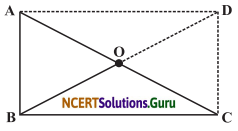# NCERT Solutions for Class 8 Maths Chapter 3 Understanding Quadrilaterals Ex 3.4

These NCERT Solutions for Class 8 Maths Chapter 3 Understanding Quadrilaterals Ex 3.4 Questions and Answers are prepared by our highly skilled subject experts.

## NCERT Solutions for Class 8 Maths Chapter 3 Understanding Quadrilaterals Exercise 3.4

Question 1.
State whether True or False.
(a) All rectangles are squares.
(b) All rhombuses are parallelograms.
(c) All squares are rhombuses and also rectangles.
(d) All squares are not parallelograms.
(e) All kites are rhombuses.
(f) All rhombuses are kites.
(g) All parallelograms are trapeziums.
(h) All squares are trapeziums.
Solution:
(a) False
(b) True
(c) True
(d) False
(e) False
(f) True
(g) True
(h) TrueQuestion 2.
Identify all the quadrilaterals that have
(a) four sides of equal length
(b) four right angles.
Solution:
(a) square, rhombus
(b) square, rectangle

Question 3.
Explain how a square is
(ii) a parallelogram
(iii) a rhombus
(iv) a rectangle
Solution:
(i) A square is a 4-sided figure, so it is a quadrilateral.
(ii) The opposite sides of a square are parallel, so it is a parallelogram.
(iii) All the sides of a square are equal, so it is a rhombus.
(iv) Each angle of a square is a right-angle, so it is a rectangle.Question 4.
(i) bisect each other
(ii) are perpendicular bisectors of each other
(iii) are equal
Solution:
(i) The diagonals of the following quadrilaterals bisect each other.
Square, rectangle, rhombus, and parallelogram
(ii) The diagonals are perpendicular bisectors of the following quadrilaterals.
Square and rhombus
(iii) The diagonals are equal in the case of square and rectangle.

Question 5.
Explain why a rectangle is a convex quadrilateral.
Solution:
A rectangle is a convex quadrilateral as both of its diagonals lies in its interior.Question 6.
ABC is a right-angled triangle and O is the mid-point of the side opposite to the right angle. Explain why O is equidistant from A, B, and C (The dotted lines are drawn additionally to help you)Solution:
Construction: Produce B to D such that BO = OD.
Proof: AO = OC (As O is a midpoint of AC)
BO = DO (By construction)
∴ ABCD is a parallelogram.
(As diagonals bisect each other)
Also, ∠B = 90°
∴ ABCD is a rectangle
∴ Diagonal AC = BD
$$\frac{1}{2}$$ AC = $$\frac{1}{2}$$ BD
AO = OB
Also, AO = OC
∴ AO = OB = OC
∴ O is equidistant from A, B and C.

error: Content is protected !!